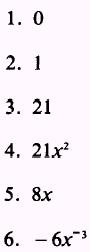Custom SearchDERIVATIVES OF VARIABLES In this section of variables, we will extend the theorems of limits covered previously. Recall that a derivative is actually a limit. The proof of the theorems presented here involve the delta process. POWER FORM Theorem 2. The derivative of the function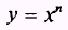is given by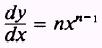if n is any real number. PROOF: By definition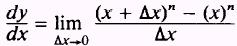The expressionmay be expanded by the binomial theorem intoSubstituting in the expression for the derivative, we have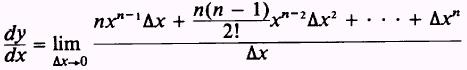Simplifying, this becomesLettingx approach zero, we haveThus, the proof is complete. EXAMPLE: Find the derivative ofSOLUTION: Apply Theorem 2, such that,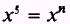Therefore, n=5 and n-1=4 so that given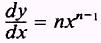and substituting values for n, find that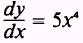EXAMPLE: Find the derivative ofSOLUTION Apply Theorem 2, such that,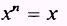Therefore,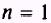and n-1=0 so that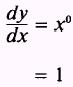The previous example is a special case of the power form and indicates that the derivative of a function with respect to itself is 1. EXAMPLE: Find the derivative of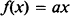where a is a constant. SOLUTIONand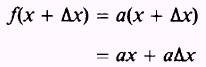so thatTherefore,Table 5-1.-Derivatives of FunctionsThe previous example is a continuation of the derivative of a function with respect to itself and indicates that the derivative of a function with respect to itself, times a constant, is that constant. EXAMPLE. Find the derivative ofSOLUTION: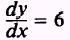A study of the functions and their derivatives in table 5-1 should further the understanding of this section. PRACTICE PROBLEMS: Find the derivatives of the following:ANSWERS: